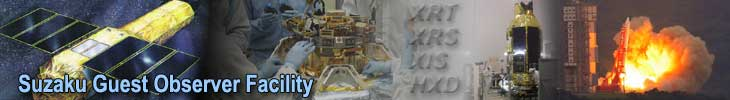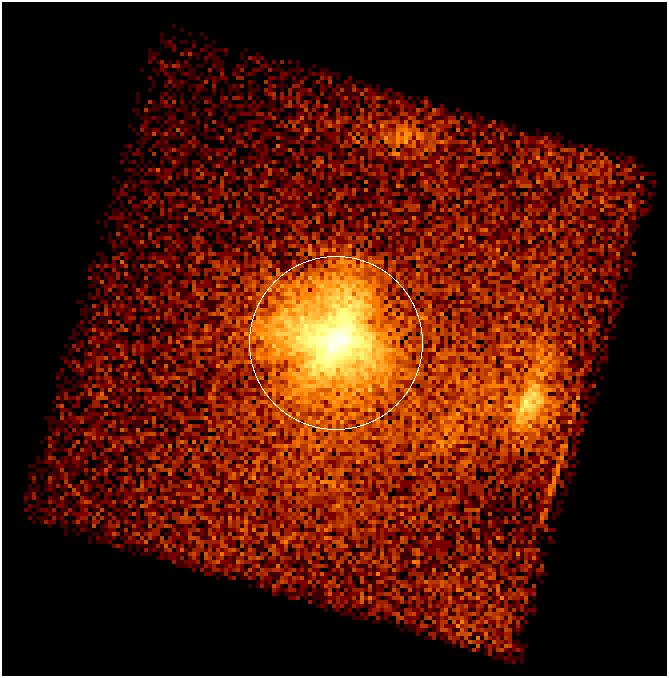## XISSIMARFGEN: Point Source Arf

Here, we show an example of generating an arf file for a point source at on-axis, using the data set ID 100012010. The following is an XIS1 image of the observation, in which one can see a bright point source at the center. We assume that a spectrum from the white encircled region in the image has been extracted, and show how to generate a corresponding ARF file.Region files with the ds9 format in the physical coordinates can be fed into xissimarfgen; when using this combination, the binning used to extract the image does not matter. To save a source region, one specifies the coordinate system "Physical" in the "File Coordinate System" row in the "Region" menu on ds9. Here is etacar_phys.reg, corresponding to the white encircled region above in the physical coordinates. Then, run the following

xissimarfgen clobber=yes \
instrume=XIS1 \
pointing=AUTO \
source_mode=J2000 \
source_ra=161.264962 \
source_dec=-59.684517 \
num_region=1 \
region_mode=SKYREG \
regfile1=etacar_phys.reg \
arffile1=xis1_etacar.arf \
limit_mode=NUM_PHOTON \
num_photon=400000 \
phafile=etacar.pi \
gtifile=100012010/xis/event_cl/ae100012010xi1_1_3x3n001_cl.evt \
attitude=100012010/auxil/ae100012010.att \
rmffile=ae_xi1_20060213.rmf \
estepfile=default

Options in black specify calibration files or Monte-Carlo simulation parameters that can be adjusted each time xissimarfgen is run, while options in red are fixed by the observation or by the upstream analysis. The "pixq_[min,max,and,eql[" parameters are not specified in the command line since we use the default setting (Bad columns, pixels, and charge injection rows are excluded; the calibration source region is not subtracted). Here we explain each option in detail.

Black options:

• The pointing option "AUTO" takes care of the referencing coordinate system (read the Recommended Usage and Caveats page for details).
• In this example, we use the limit_mode option "NUM_PHOTON" and set the number of simulated photons (num_photon) of 400,000, to minimize the Poisson noise in the ARF.
• Through spectrum passed using the phafile parameter, xissimarfgen knows the XIS observing mode such as the window option and spaced-row charge injection option.
• In this example, we do not use the detmask option since the calibration source events do not fall on the source region.
• The "default" selection for the estepfile option has a sufficient energy resolution for most purposes.

Red options:

• The instrume option is XIS1, set by the spectrum being analyzed.
• In this example, we specify the source position in the J2000 coordinate system. Thus, the source_mode is "J2000." Therefore we also input the source coordinates [(R.A., Dec.)(J2000) = (161.264962, -59.684517)] using the source_ra and source_dec parameters. See the example below for using the physical (X, Y) coordinate.
• The num_region option should be "1" since, in this case, we generate only one ARF file. The region_mode option is SKYREG since the region file is described in the sky coordinate system. The region file name, "etacar_phys.reg", is specified in the regfile1 parameter.
• The output ARF file name "xis1_etacar.arf" is specified using the arffile1 parameter.
• To take the attitude wobble into account, the attitude file in the auxil directory, is loaded using the attitude parameter. To specify the time span of the observation, a FITS file with the GTI table of the observation ("ae100012010xi1_1_3x3n001_cl.evt" in this case) is loaded using the gtifile parameter.
• We also specify the RMF to be used in spectral fitting (ae_xi1_20060213c.rmf in this case).
Here is an example, in which the source position is specified in the SKYXY coordinate.

xissimarfgen clobber=yes \
instrume=XIS1 \
pointing=AUTO \
source_mode=SKYXY \
source_x=784.5 \
source_y=786.5 \
num_region=1 \
region_mode=SKYREG \
regfile1=etacar_phys.reg \
arffile1=xis1_etacar.arf \
limit_mode=NUM_PHOTON \
num_photon=400000 \
phafile=none \